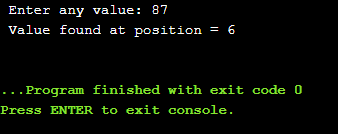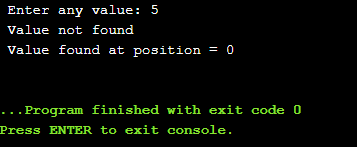C++ Programming

# Searching in C++: Sequential Searching and Binary Searching

## Searching in one-dimensional array:

Searching in C++, this is the process of finding a certain data item from a given list of values, this process is called searching. The searching process is considered successful if the specified data item is successfully found during the searching process in C++ otherwise, if the specified data item is not found then the result is declared unsuccessful. The search operation comes to an end or is terminated when the specified item is found. This may also be possible that more than one instance of the searched item may be present in the provided list.

A variety of search methods can be used (depending on the situation) for searching information. The most commonly used types of the searches methods are given below.

• Sequential search and
• Binary search

Top Gaming Computers

Best Laptops

Best Graphic Cards

Portable Hard Drives

Best Keyboards

Best High Quality PC Mic

Computer Accessories

## Sequential Search:

Sequential search is also known as linear search or serial. The Sequential search method is a very simple and straightforward technique to search a specified data item in an unordered list. The data to be found is searched in the list sequentially, i.e. starting from the first data item up to the required data item of the list in a sequence.

Using sequential search, the following procedure is adopted:

• The desired search value is compared with the first value in the list. If the required value matches with the first value, the search operation is declared successful and is stops. Suppose we want to search value 63 in the list of value as shown in the figure given below. This value exists at location 6 and 9. The search operation is terminated at position 6.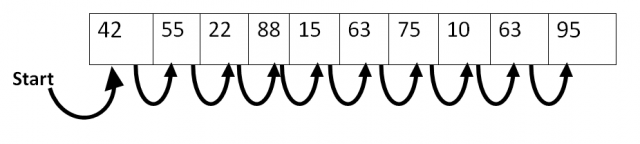• If the desired value doesn’t match with the first value of the list, it is compared with the second value. The search cycle proceeds till the value is found or end of the list is reached. Assume we need to search 66 in the list of values as appeared in figure. This value doesn’t exist in the list as given underneath. The search operation is ended toward the end of the list and is terminated.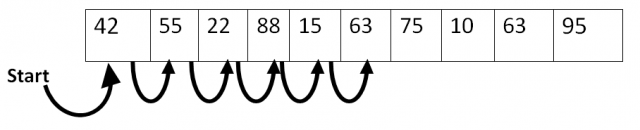The sequential search is slow and is used for an only a small list of data. This method is not recommended for a large amount of data because some more efficient method is available for large and complex search.

### Example: how to use sequential searching in C++ for search a value in an array list and display their position on the screen:

```#include <iostream>
using namespace std;

int main()
{
int arr, n, i, pos;
i=0;
while(i<=4)
{
cout<<" enter value in element "<<i<<" :";
cin>>arr[i];
i++;

}
pos = 0;
cout<<" enter any value :";
cin>>n;
i=0;
while(i<=5)
{

if(n==arr[i])
{

pos=i+1;
break;

}
i++;

}
if(pos==0)
else
cout<<" Value found at position = "<<pos<<endl;
}
```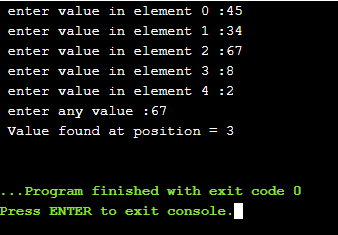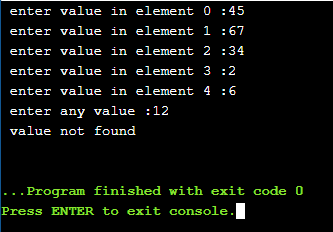### Example: how to find maximum value and its location in the array using sequential Searching in C++:

```#include <iostream>

using namespace std;

int main()
{
float arr, max;
int loc, i=0;
while(i<=9)
{
cout<<" enter value in element "<<i<<" :";
cin>>arr[i];
i++;

}
max=arr;
i=1;
while(i<=9)
{
if(max<arr[i])
{
max = arr[i];
loc=i;

}
i++;

}

cout<<"\nMaximum value is :"<<max<<endl;
cout<<"Location of the value in array :"<<loc+1;

}
```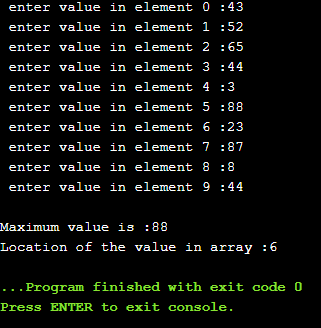## Binary Searching in C++:

The Binary searching technique is used to search the desired data value or item in an ordered list(i.e the values sorted in ascending or descending order). In C++ as compared to the Sequential searching the binary Searching in C++ is very fast. It is used to search large-size list to find a specific value.

In binary searching, the search process is started from the middle of the sorted list. If the required value is in the middle of the list then searching process is successful and is stopped at that point; otherwise one of two halves of the list is selected for further Searching in C++. The main purpose of the binary search is to repeatedly cut a given list into two halves with every comparison.

Suppose an array “abc” with 10 values in sorted order as shown in the following figure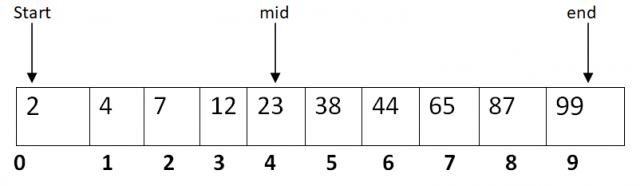The following steps explain the binary Searching in C++ for finding a specific value in the above array. Suppose we want to search the value 12 in the above array.

Step-1:

Divide the array into two halves such as mid =(start + end)/2. In the above case, the value of start is 0, while the end is 9. So the value of mid is 4. The value mid (i.e. 23) is greater than 12. The required value (i.e. 12) exists on the left half side of the array, i.e. 0 to 3.

Step-2:

Divide again left side of the array from 0 to 3. In this case, new values of start and end are 0 and 3 (end = mid-1) respectively. Mid = (start + end)/2. So the new value of mid is 1. The value of mid (i.e. 4) is less than 12. The required value (i.e. 12 ) exists on the right half side, i.e. 2 to 3.

Step-3:

Divide again array from 2 to 3. In this case, new values of start and end are 2 and 3 (start = mid +1) respectively. Mid = (start+end)/2. So the new value of mid is 2. The value of mid (i.e. 7) is less than 12. The required value (i.e. 12) exists on the right half side, i.e. 3 to 3.

Step-4:

Divide again array from 3 to 3. In this case, new values of start and end are 3 and 3 (start = mid + 1) respectively. Mid = (start + end)/2. So the new values of mid is 3. The value of mid (i.e. 12) is equal to 12.t the new value is located at position3. The search process is terminated.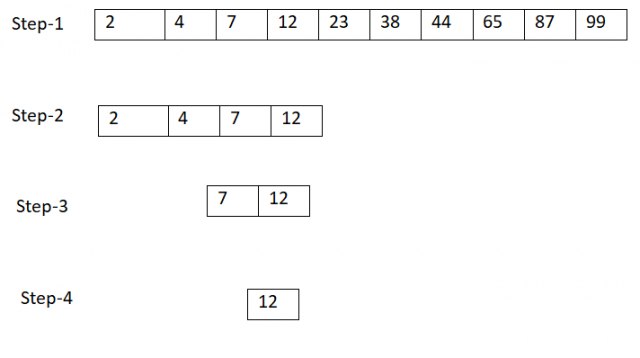### Example: write a program that initialize data into one-dimensional array and searches the value in the array using binary searching in c++:

```#include <iostream>
using namespace std;

int main()
{
int abc={1,3,9,15,78,87,95,103,124,352};
int loc, num, i, m, s=0,e=9;
loc=0;
cout<<" Enter any value: ";
cin>>num;
while(s<e)
{
m=(s+e)/2;
if(num==abc[m])
{
loc= m+1;
break;

}
else if(num<abc[m])
e=m-1;
else
s=m+1;

}
if(loc==0)
cout<<" Value found at position = "<<loc<<endl;

}
```